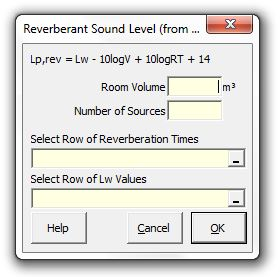### Strutt Help

Reverberant Sound Level (from RT)    1/1, 1/3

Strutt|Building Acoustics|Reverberant Sound Level (from RT) inserts the reverberant sound pressure level into the active row of the worksheet.The reverberant sound pressure level is calculated using the equation:

L_(p,rev) = L_W - 10log_10(V) + 10 log_10(T) + 14

Where:
L_W is the source sound power level (dB)
V is the room volume (m3)
T is the room reverberation time

Note that this approach is an approximation for situations where the average room absorption coeffient alpha_(ave) is low; for cases where the average room absorption is high, consider using the Reverberant Level (From Room Constant) calculation instead.

References:

• Arup Sound and Vibration in Mechanical Services Design, p28